##Cross Ratio

Given a line (e) and four points A, B, C, and D on it, the cross ratio of these four points is defined by the equation:
(ABCD) = ((a-c)/(b-c))/((a-d)/(b-d)).
The right side of this equation is defined as the cross ratio of four real numbers. Here we identify the point with its line coordinate relative to an arbitrary coordinate system of the line. i.e. fixing two points O, E on the line we measure the location of an arbitrary point X by the number x = OX/OE (may be negative). Thus points A(a), B(b), C(c) and D(d) define the respective coordinates a,b,c and d.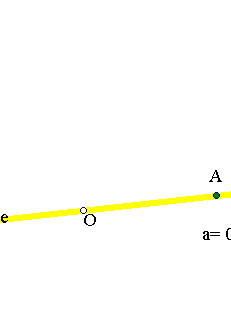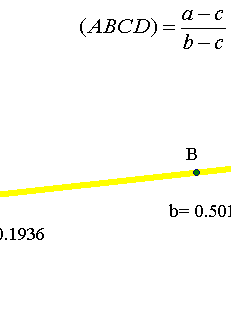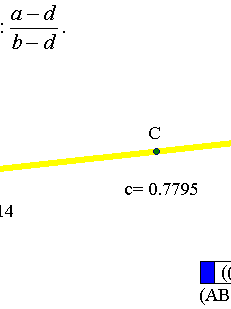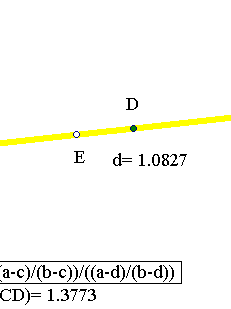The definition is independend of the location of O and E i.e. independent of the special line coordinate system. In fact, changing the coordinate system to some other (defined by two other O', E'), the new coordinate x' is related to the old by a relation of the form x = H(x) = m*x'+n, m and n being appropriate constants. The clue is that by substituting this expression of x into the formula we get the same number expressed in the other coordinates i.e.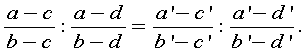More generally the cross ratio of four numbers (abcd) = ((a-c)/(b-c))/((a-d)/(b-d)) is invariant under the broken linear functions x = h(x) = (m*x'+n)/(p*x'+q) and this makes it possible to generalize the cross ratio for four points A, B, C and D lying on a conic (see CrossRatio.html ).
Relations between two variables of the above form: x = h(x) = (m*x'+n)/(p*x'+q), with non-zero determinant (m*q-n*p) are called homographic relations and are studied in HomographicRelation.html .
The definition allows for a number to be taken at infinity. F.e. taking d at infinity the cross ratio reduces to (a-c)/(b-c). Taking further c=0, this amounts to a/b. Thus, the ratio of two numbers is the cross ratio (ab0I), I standing for infinity.
It is astonishing how many geometric facts depend on the cross-ratio and its properties. In fact, it can be proved that the cross-ratio, considered as a function of four points (variables), is the unique invariant of the projective geometry. Hence one can expect to find it behind every incidence relation between points and lines. This is verified f.e. in the case of Pascal's theorem (see Pascal.html ).
Below is given a list of properties of the cross ratio.

##Properties of the cross-ratio of four real numbers

 Given 3 pairwise different real numbers x1, x2, x3 the function y=f(x) = (x1x2x3x) defines an invertible homographic relation, whose graph is a rectangular hyperbola.
 Given three pairs of real numbers (x1,y1), (x2,y2), (x3,y3) in general position equation (y1y2y3y) = (x1x2x3x) defines the unique homographic relation y=f(x), such that f(x1)=y1, f(x2)=y2, f(x3)=y3.
 Every permutation which is product of two transpositions of the letters a,b,c,d leaves the cross ratio invariant i.e.
(abcd) = (badc) = (cdab) = (dcba). Hence from the 4! in total permutations of the 4 letters, only 6 give different values.
 (abdb) = (abcd)-1 and (acbd)+(abcd)=1. If follows that:
 If (abcd) = k, then
(abdc) = k-1,
(acbd) = 1-k,
(acdb) = 1/(1-k),
 The four numbers a,b,c,d are pairwise different if and only if (abcd) has a value different from 1, 0 and I (infiinity).
 In addition to these formulas, important formulas arise in the case (abcd) = -1 i.e. when the numbers build a harmonic set of points (see Harmonic.html ).

##Basic property of cross-ratio of four points

Take four points A, B, C, D on a line (e) and a fifth point E outside (e). Then the cross ratio has an interpretation in terms of the angles at E of lines EA, EB, EC, ED. We speak about the bundle of lines at E and denote with E(ABCD) the corresponding ratio. The dependence of E(ABCD) from the angles only shows that a second line (g) inersecting the four lines EA, EB, EC, ED defines the same cross ratio.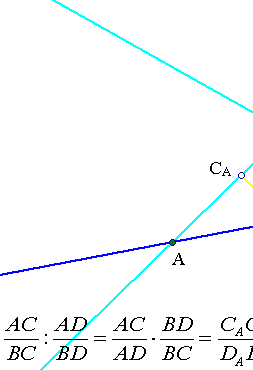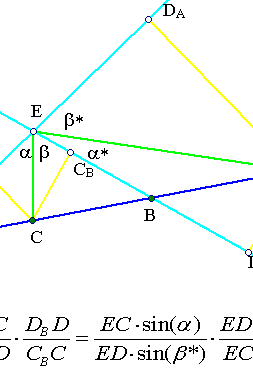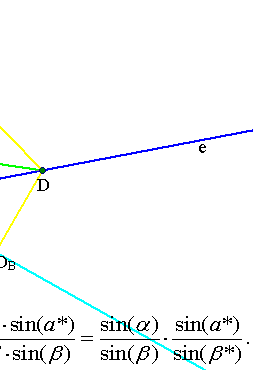See the file CrossRatioLines.html for the version of cross ratio for a bundle of four concurring lines.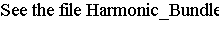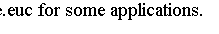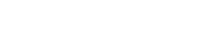Chasles_Steiner.html
Chasles_Steiner_Envelope.html
Complex_Cross_Ratio.html
Complex_Cross_Ratio2.html
CrossRatio.html
CrossRatioLines.html
FourTangentsCrossRatio.html GoodParametrization.html
Harmonic.html
Harmonic_Bundle.html